Next: Approximate methods and standard Up: Uncertainties from systematic effects Previous: Joint inference and marginalization

## Correlation in results caused by systematic errors

We can easily extend Eqs. (73), (77), and (79) to a joint inference of several variables, which, as we have seen, are nothing but parameters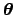of suitable models. Using the alternative ways described in Sects. 6.1 and 6.2, we have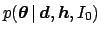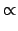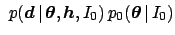(80)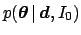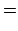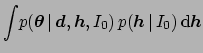(81)

and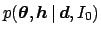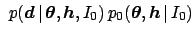(82)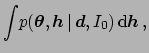(83)

respectively. The two ways lead to an identical result, as it can be seen comparing Eqs. (81) and (83).

Take a simple case of a common offset error of an instrument used to measure various quantities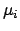, resulting in the measurements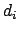. We model each measurement asplus an error that is Gaussian distributed with a mean of zero and a standard deviation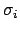. The calculation of the posterior distribution can be performed analytically, with the following results (see D'Agostini 1999c for details):

• The uncertainty in eachis described by a Gaussian centered at, with standard deviation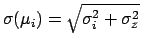, consistent with Eq. (76).
• The joint posterior distribution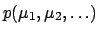does not factorize into the product of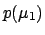,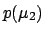, etc., because correlations are automatically introduced by the formalism, consistent with the intuitive thinking of what a common systematic should do. Therefore, the joint distribution will be a multi-variate Gaussian that takes into account correlation terms.
• The correlation coefficient between any pair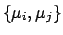is given by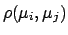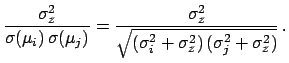(84)

We see thathas the behavior expected from a common offset error; it is non-negative; it varies from practically zero, indicating negligible correlation, when (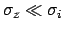), to unity (), when the offset error dominates.Next: Approximate methods and standard Up: Uncertainties from systematic effects Previous: Joint inference and marginalization
Giulio D'Agostini 2003-05-13